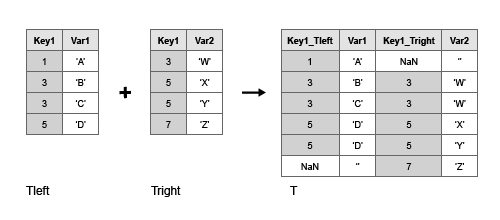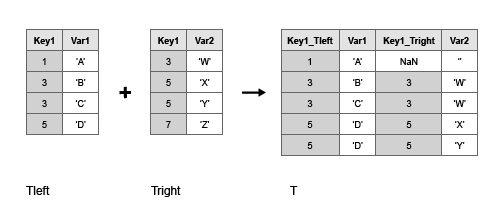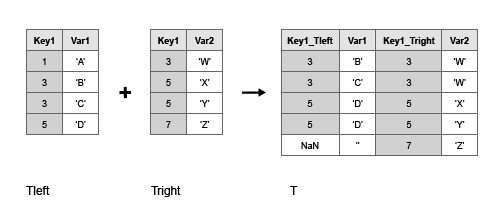Main Content

# outerjoin

Outer join between two tables or timetables

## Syntax

``T = outerjoin(Tleft,Tright)``
``T = outerjoin(Tleft,Tright,Name,Value)``
``````[T,ileft,iright] = outerjoin(___)``````

## Description

example

````T = outerjoin(Tleft,Tright)` creates the table or timetable, `T`, as the outer join of `Tleft` and `Tright` using key variables. All variables with the same names in both tables are key variables. An outer join combines table rows where the key variables have matching values, but it also includes rows where the key variables from one input table have no matches in the other input table. For example, if `Tleft` has variables named `Key1` and `Var1`, and `Tright` has variables `Key1` and `Var2`, then `T=outerjoin(Tleft,Tright)` uses `Key1` as a key variable.The matching values of the key variables in the left and right tables do not have to be in the same order. Outer joins can perform one-to-many and many-to-one matches between the key variables of the two tables. That is, a value that occurs once in a key variable of the left table can have multiple matches in the right table. Similarly, a value that occurs once in a key variable of the right table can have multiple matches in the left table.You can perform outer joins only on certain combinations of tables and timetables.If `Tleft` is a table, then `Tright` must be a table. `outerjoin` returns `T` as a table.If `Tleft` is a timetable, then `Tright` can be either a table or a timetable. `outerjoin` returns `T` as a timetable for either combination of inputs.The vectors of row labels of `Tleft` and `Tright` can be key variables. Row labels are the row names of a table, or the row times of a timetable.```

example

````T = outerjoin(Tleft,Tright,Name,Value)` performs the outer-join operation with additional options specified by one or more `Name,Value` pair arguments.```

example

``````[T,ileft,iright] = outerjoin(___)``` also returns index vectors, `ileft` and `iright`, indicating the correspondence between rows in `T` and rows in `Tleft` and `Tright` respectively. You can use this syntax with any of the input arguments in the previous syntaxes.```

## Examples

collapse all

Create a table, `Tleft`.

```Tleft = table([5;12;23;2;15;6],... {'cheerios';'pizza';'salmon';'oreos';'lobster';'pizza'},... 'VariableNames',{'Age','FavoriteFood'},... 'RowNames',{'Amy','Bobby','Holly','Harry','Marty','Sally'})```
```Tleft=6×2 table Age FavoriteFood ___ ____________ Amy 5 {'cheerios'} Bobby 12 {'pizza' } Holly 23 {'salmon' } Harry 2 {'oreos' } Marty 15 {'lobster' } Sally 6 {'pizza' } ```

Create a table, `Tright`, with one variable in common with `Tleft`, called `FavoriteFood`.

```Tright = table({'cheerios';'oreos';'pizza';'salmon';'cake'},... [110;160;140;367;243],... {'A-';'D';'B';'B';'C-'},... 'VariableNames',{'FavoriteFood','Calories','NutritionGrade'})```
```Tright=5×3 table FavoriteFood Calories NutritionGrade ____________ ________ ______________ {'cheerios'} 110 {'A-'} {'oreos' } 160 {'D' } {'pizza' } 140 {'B' } {'salmon' } 367 {'B' } {'cake' } 243 {'C-'} ```

Use the `outerjoin` function to create a new table, `T`, with data from tables `Tleft` and `Tright`.

`T = outerjoin(Tleft,Tright)`
```T=7×5 table Age FavoriteFood_Tleft FavoriteFood_Tright Calories NutritionGrade ___ __________________ ___________________ ________ ______________ NaN {0x0 char } {'cake' } 243 {'C-' } 5 {'cheerios'} {'cheerios'} 110 {'A-' } 15 {'lobster' } {0x0 char } NaN {0x0 char} 2 {'oreos' } {'oreos' } 160 {'D' } 12 {'pizza' } {'pizza' } 140 {'B' } 6 {'pizza' } {'pizza' } 140 {'B' } 23 {'salmon' } {'salmon' } 367 {'B' } ```

Table `T` contains a separate variable for the key variable from `Tleft`, called `FavoriteFood_Tleft`, and the key variable from `Tright`, called `FavoriteFood_Tright`.

Create a table, `Tleft`.

```Tleft = table({'a' 'b' 'c' 'e' 'h'}',[1 2 3 11 17]',... 'VariableNames',{'Key1' 'Var1'})```
```Tleft=5×2 table Key1 Var1 _____ ____ {'a'} 1 {'b'} 2 {'c'} 3 {'e'} 11 {'h'} 17 ```

Create a table, `Tright`, with common values in the variable `Key1` between tables `Tleft` and `Tright`, but also containing rows with values of `Key1` not present in `Tleft`.

```Tright = table({'a','b','d','e'}',[4;5;6;7],... 'VariableNames',{'Key1' 'Var2'})```
```Tright=4×2 table Key1 Var2 _____ ____ {'a'} 4 {'b'} 5 {'d'} 6 {'e'} 7 ```

Use the `outerjoin` function to create a new table, `T`, with data from tables `Tleft` and `Tright`. Merge the key values into a single variable in the output table, `T`.

`T = outerjoin(Tleft,Tright,'MergeKeys',true)`
```T=6×3 table Key1 Var1 Var2 _____ ____ ____ {'a'} 1 4 {'b'} 2 5 {'c'} 3 NaN {'d'} NaN 6 {'e'} 11 7 {'h'} 17 NaN ```

Variables in table `T` that came from `Tleft` contain null values in the rows that have no match from `Tright`. Similarly, variables in `T` that came from `Tright` contain null values in those rows that had no match from `Tleft`.

Create a table, `Tleft`.

```Tleft = table({'a' 'b' 'c' 'e' 'h'}',[1 2 3 11 17]',... 'VariableNames',{'Key1' 'Var1'})```
```Tleft=5×2 table Key1 Var1 _____ ____ {'a'} 1 {'b'} 2 {'c'} 3 {'e'} 11 {'h'} 17 ```

Create a table, `Tright`, with common values in the variable `Key1` between tables `Tleft` and `Tright`, but also containing rows with values of `Key1` not present in `Tleft`.

```Tright = table({'a','b','d','e'}',[4;5;6;7],... 'VariableNames',{'Key1' 'Var2'})```
```Tright=4×2 table Key1 Var2 _____ ____ {'a'} 4 {'b'} 5 {'d'} 6 {'e'} 7 ```

Use the `outerjoin` function to create a new table, `T`, with data from tables `Tleft` and `Tright`. Match up rows with common values in the key variable, `Key1`, but also retain rows whose key values don’t have a match.

Also, return index vectors, `ileft` and `iright` indicating the correspondence between rows in `T` and rows in `Tleft` and `Tright` respectively.

`[T,ileft,iright] = outerjoin(Tleft,Tright)`
```T=6×4 table Key1_Tleft Var1 Key1_Tright Var2 __________ ____ ___________ ____ {'a' } 1 {'a' } 4 {'b' } 2 {'b' } 5 {'c' } 3 {0x0 char} NaN {0x0 char} NaN {'d' } 6 {'e' } 11 {'e' } 7 {'h' } 17 {0x0 char} NaN ```
```ileft = 6×1 1 2 3 0 4 5 ```
```iright = 6×1 1 2 0 3 4 0 ```

The index vectors `ileft` and `iright` contain zeros to indicate the rows in table `T` that do not correspond to rows in tables `Tleft` or `Tright`, respectively.

Create a table, `Tleft`.

```Tleft = table({'a' 'b' 'c' 'e' 'h'}',[1 2 3 11 17]',... 'VariableNames',{'Key1' 'Var1'})```
```Tleft=5×2 table Key1 Var1 _____ ____ {'a'} 1 {'b'} 2 {'c'} 3 {'e'} 11 {'h'} 17 ```

Create a table, `Tright`, with common values in the variable `Key1` between tables `Tleft` and `Tright`, but also containing rows with values of `Key1` not present in `Tleft`.

```Tright = table({'a','b','d','e'}',[4;5;6;7],... 'VariableNames',{'Key1' 'Var2'})```
```Tright=4×2 table Key1 Var2 _____ ____ {'a'} 4 {'b'} 5 {'d'} 6 {'e'} 7 ```

Use the `outerjoin` function to create a new table, `T`, with data from tables `Tleft` and `Tright`. Ignore rows in `Tright` whose key values do not match any rows in `Tleft`.

Also, return index vectors, `ileft` and `iright` indicating the correspondence between rows in `T` and rows in `Tleft` and `Tright` respectively.

`[T,ileft,iright] = outerjoin(Tleft,Tright,'Type','left')`
```T=5×4 table Key1_Tleft Var1 Key1_Tright Var2 __________ ____ ___________ ____ {'a'} 1 {'a' } 4 {'b'} 2 {'b' } 5 {'c'} 3 {0x0 char} NaN {'e'} 11 {'e' } 7 {'h'} 17 {0x0 char} NaN ```
```ileft = 5×1 1 2 3 4 5 ```
```iright = 5×1 1 2 0 4 0 ```

All values of `ileft` are nonzero indicating that all rows in `T` have corresponding rows in `Tleft`.

Create two timetables, `Tleft` and `Tright`. They have some row times in common, but each also includes row times that are not in the other timetable.

`Tleft = timetable(seconds([1;2;4;6]),[1 2 3 11]')`
```Tleft=4×1 timetable Time Var1 _____ ____ 1 sec 1 2 sec 2 4 sec 3 6 sec 11 ```
`Tright = timetable(seconds([2;4;6;7]),[4 5 6 7]')`
```Tright=4×1 timetable Time Var1 _____ ____ 2 sec 4 4 sec 5 6 sec 6 7 sec 7 ```

Combine `Tleft` and `Tright` with an outer join. `T1` matches up the rows with common row times, but also includes the rows that do not have matches.

`T1 = outerjoin(Tleft,Tright)`
```T1=5×2 timetable Time Var1_Tleft Var1_Tright _____ __________ ___________ 1 sec 1 NaN 2 sec 2 4 4 sec 3 5 6 sec 11 6 7 sec NaN 7 ```

Combine `Tleft` and `Tright`, but ignore rows in `Tright` whose row times do not match any row times in `Tleft`.

`T2 = outerjoin(Tleft,Tright,'Type','left')`
```T2=4×2 timetable Time Var1_Tleft Var1_Tright _____ __________ ___________ 1 sec 1 NaN 2 sec 2 4 4 sec 3 5 6 sec 11 6 ```

## Input Arguments

collapse all

Left table, specified as a table or a timetable.

Right table, specified as a table or a timetable.

### Name-Value Pair Arguments

Specify optional comma-separated pairs of `Name,Value` arguments. `Name` is the argument name and `Value` is the corresponding value. `Name` must appear inside quotes. You can specify several name and value pair arguments in any order as `Name1,Value1,...,NameN,ValueN`.

Example: `'Keys',2` uses the second variable in `Tleft` and the second variable in `Tright` as key variables.

Variables to use as keys, specified as the comma-separated pair consisting of `'Keys'` and a positive integer, vector of positive integers, character vector, cell array of character vectors, string array, or logical vector.

You cannot use the `'Keys'` name-value pair argument with the `'LeftKeys'` and `'RightKeys'` name-value pair arguments.

A vector of row labels can be a key, alone or in combination with other key variables. For more information, see the Tips section.

Example: `'Keys',[1 3]` uses the first and third variables in `Tleft` and `Tright` as key variables.

Example: `'Keys',{'X','Y'}` uses the variables named `X` and `Y` in `Tleft` and `Tright` as key variables.

Example: `'Keys','Row'` uses the vectors of row names of `Tleft` and `Tright` as key variables, if both `Tleft` and `Tright` are tables with row names.

Variables to use as keys in `Tleft`, specified as the comma-separated pair consisting of `'LeftKeys'` and a positive integer, vector of positive integers, character vector, cell array of character vectors, string array, or logical vector.

You must use the `'LeftKeys'` name-value pair argument in conjunction with the `'RightKeys'` name-value pair argument. `'LeftKeys'` and `'RightKeys'` both must specify the same number of key variables. `outerjoin` pairs key values based on their order.

A vector of row labels can be a key, alone or in combination with other key variables. For more information, see the Tips section.

Example: `'LeftKeys',1` uses only the first variable in `Tleft` as a key variable.

Variables to use as keys in `Tright`, specified as the comma-separated pair consisting of `'RightKeys'` and a positive integer, vector of positive integers, character vector, cell array of character vectors, string array, or logical vector.

You must use the `'RightKeys'` name-value pair argument in conjunction with the `'LeftKeys'` name-value pair argument. `'LeftKeys'` and `'RightKeys'` both must specify the same number of key variables. `outerjoin` pairs key values based on their order.

A vector of row labels can be a key, alone or in combination with other key variables. For more information, see the Tips section.

Example: `'RightKeys',3` uses only the third variable in `Tright` as a key variable.

Merge keys flag, specified as the comma-separated pair consisting of `'MergeKeys'` and either `false`, `true`, `0` or `1`.

 `false` `outerjoin` includes two separate variables in the output table, `T`, for each key variable pair from tables `Tleft` and `Tright`.This behavior is the default behavior. `true` `outerjoin` includes a single variable in the output table, `T`, for each key variable pair from tables `Tleft` and `Tright`.`outerjoin` creates the single variable by merging the key values from `Tleft` and `Tright`, taking values from `Tleft` where a corresponding row exists in `Tleft`, and taking values from `Tright` otherwise.If you specify `'LeftVariables'` or `'RightVariables'` to include only one key from a key variable pair, then `outerjoin` includes the merged key—containing values from both key variables—in the output table.If you specify `'LeftVariables'` and `'RightVariables'` to exclude both keys from a key variable pair, then `outerjoin` does not include the merged key variable in the output table.

Variables from `Tleft` to include in `T`, specified as the comma-separated pair consisting of `'LeftVariables'` and a positive integer, vector of positive integers, character vector, cell array of character vectors, string array, or logical vector.

You can use `'LeftVariables'` to include or exclude key variables as well as nonkey variables from the output, `T`.

By default, `outerjoin` includes all variables from `Tleft`.

Variables from `Tright` to include in `T`, specified as the comma-separated pair consisting of `'RightVariables'` and a positive integer, vector of positive integers, character vector, cell array of character vectors, string array, or logical vector.

You can use `'RightVariables'` to include or exclude key variables as well as nonkey variables from the output, `T`.

By default, `outerjoin` includes all the variables from `Tright`.

Type of outer join operation, specified as the comma-separated pair consisting of `'Type'` and either `'full'` (meaning a full outer join), `'left'` (left outer join), or `'right'` (right outer join).

• For a full outer join, `T` includes all key values from both tables, as shown in the diagram below.• For a left outer join, `T` includes key values in `Tleft` that do not match any key values in `Tright`, but excludes key values in `Tright` that do not match any key values in `Tleft`. For example, in the diagram below, the left outer join excludes the key value `7` from the right table.• For a right outer join, `T` includes key values in `Tright` that do not match any key values in `Tleft`, but excludes key values in `Tleft` that do not match any key values in `Tright`. For example, in the diagram below, the right outer join excludes the key value `1` from the left table.By default, `outerjoin` does a full outer join and includes unmatched rows from both `Tleft` and `Tright`.

## Output Arguments

collapse all

Outer join from `Tleft` and `Tright`, returned as a table. The output table, `T`, contains one row for each pair of rows in tables `Tleft` and `Tright` that share the same combination of key values. If `Tleft` and `Tright` contain variables with the same name, `outerjoin` adds a unique suffix to the corresponding variable names in `T`. Variables in `T` that came from `Tleft` contain null values in those rows that had no match from `Tright`. Similarly, variables in `T` that came from `Tright` contain null values in those rows that had no match from `Tleft`.

In general, if there are `m` rows in table `Tleft` and `n` rows in table `Tright` that all contain the same combination of values in the key variables, table `T` contains `m*n` rows for that combination. `T` also contains rows corresponding to key value combinations in one input table that do not match any row the other input table.

`T` contains the horizontal concatenation of `Tleft(ileft,LeftVars)` and `Tright(iright,RightVars)` sorted by the values in the key variables. By default, `LeftVars` consists of all the variables of `Tleft`, and `RightVars` consists of all the variables from `Tright`. Otherwise, `LeftVars` consists of the variables specified by the `'LeftVariables'` name-value pair argument, and `RightVars` consists of the variables specified by the `'RightVariables'` name-value pair argument.

You can store additional metadata such as descriptions, variable units, variable names, and row names in the table. For more information, see the Properties section of `table`.

Index to `Tleft`, returned as a column vector. Each element of `ileft` identifies the row in table `Tleft` that corresponds to that row in the output table, `T`. The vector `ileft` contains zeros to indicate the rows in `T` that do not correspond to rows in `Tleft`.

Index to `Tright`, returned as a column vector. Each element of `iright` identifies the row in table `Tright` that corresponds to that row in the output table, `T`. The vector `iright` contains zeros to indicate the rows in `T` that do not correspond to rows in `Tright`.

## More About

collapse all

### Key Variable

Variable used to match and combine data between the input tables, `Tleft` and `Tright`.

## Tips

• The vector of row labels from an input table or timetable can be a key, alone or in combination with other key variables. Row labels are the row names of a table or the row times of a timetable. To use this vector as a key, specify it as `'Row'` (for the row names of a table), as the name of a timetable vector of row times, or as the value of `T.Properties.DimensionNames{1}`, where `T` is the table or timetable.

In general, `outerjoin` copies row labels from the input table `Tleft` to the output table `T`.

• If `Tleft` has no row labels, then `T` has no row labels.

• If `Tleft` has row labels, then `outerjoin` copies row labels from `Tleft` to create row labels in `T`.

• If you specify row labels from both `Tleft` and `Tright` as a key pair, then `outerjoin` merges row labels from `Tright` into row labels of `T` where needed.

• If you specify row labels of `Tleft` as a key, but do not specify row labels of `Tright` as the matching key, then `outerjoin` creates default row labels in `T` where needed.

• If both `Tleft` and `Tright` are tables, but you do not specify either input table’s row names as a key, then `outerjoin` does not create row names in `T`.

You cannot perform an outer join using the row labels of `Tleft` as the left key and a variable of `Tright` as the right key. To perform the outer join, convert the row labels of `Tleft` to a table variable and use the new table variable as a key.

## See Also

Introduced in R2013b

Download ebook